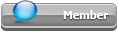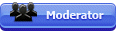## Featured Articles

Check out the latest featured articles.## File Library# Specific Heat Of Natural Gas

This topic has been archived. This means that you cannot reply to this topic.
7 replies to this topic
|

### #1•• guestGuests
• 0 posts

Posted 06 April 2009 - 08:40 AM

Please help me in working out the specific heat of gas at different operating pressure and temperature.

Thanks

### #2Zauberberg

Zauberberg

Gold Member

•• ChE Plus Subscriber
•• 2,715 posts

Posted 06 April 2009 - 09:29 AM

One of the resources you may look into, is "The properties of gases and liquids" by J.M. Prausnitz. This is considered as "classic" amongst ChE's.

Another option is to use simulator software with appropriate thermo package.

### #3ankur2061

ankur2061

Gold Member

•• Forum Moderator
•• 2,484 posts

Posted 06 April 2009 - 10:15 AM

Avtar,

Your question is very general and does not specify what you are actually looking for. Are you interested to know Cp (specific heat at constant pressure) or Specific heat at constant volume (Cv)? For engineering applications related to gas compression and expansion the useful engineering entity is specific heat ratio (k) also known as isentropic expansion coefficient and is defined as:

k = Cp/Cv

Specific heat at constant pressure (Cp) of pure gases is readily available from a wide source available in physics, chemistry or thermodynamics textbooks and even on the internet as a free resource. One such free, recognized and reliable resource can be found as below:

http://webbook.nist.gov/chemistry/

If you are dealing with a gas mixture then the Cp of the gas mixture can be found out by the law of proportion based on the mole fraction of the individual pure components of the gas. The Cp of the gas mixture of say A,B & C components thus would be:

mole fraction of A*Cp of A + mole fraction of B*Cp of B + mole fraction of C*Cp of C

The major obstacle to finding k is the non-availability of Cv values. However for gases or gas mixtures where the behaviour approximates that of an ideal gas you can calculate k by the following equation:

k = Cp/(Cp-(8.314/MW))

where Cp = specific heat at constant pressure, kJ/kg-K
MW = molecular weight of the gas or gas mixture

Although the above equation is applicable for ideal gases for most engineering calculations involving k value for compression and expansion this is adequate.

Experimentally derived k values for gases (e.g. air) are the most accurate data and should be used whenever available for engineering calculations. k values generated by simulation software such as HYSYS are fairly accurate and should be used whenever available since these values can be generated at the actual pressure and temperature conditions approximating real conditions and using equations of state such as Peng-Robinson (PR) and Soave-Redlich-Kwong (SRK).

I could also find an equation for calculating k for gases approximating real behaviour which is as follows:

k = Cp/Cv = Cp/Cp-R [1 + (Pr/Tr2)[0.132+0.712/Tr2]

where

Tr = reduced temperature of the gas or gas mixture
Pr = reduced pressure of the gas or gas mixture
Cp = specific heat at constant presure of the gas or gas mixture
R= universal gas constant

Use consistent set of units

The above mentioned equation can be found in the March 14, 1977 edition of 'Chemical Engineering' magazine.

Further reading for specific heat ratio definition, equation and usage can be found at:

http://en.citizendiu...ific_heat_ratio

http://en.wikipedia...._capacity_ratio

Regards,
Ankur.

### #4kuldeepd

kuldeepd

Junior Member

•• Members
•• 19 posts

Posted 14 April 2009 - 12:09 AM

For calculations, this may help
http://www.processca...cific_Heat.aspx

### #5ankur2061

ankur2061

Gold Member

•• Forum Moderator
•• 2,484 posts

Posted 13 June 2009 - 08:38 AM

Dear All,

Please find attached a spreadsheet for calculation of 'Heat Capacity Ratio of Real Gases'. Comments and queries are most welcome.

Regards,
Ankur.

### #6HK1234

HK1234

Brand New Member

•• Members
•• 4 posts

Posted 11 April 2012 - 02:34 AM

I'm new here. That's a very useful spreadsheet. Many thanks, Ankur.

### #7dnoel

dnoel

Brand New Member

•• Members
•• 1 posts

Posted 20 January 2013 - 03:22 PM

Ankur, thanks for your information given in this post. Just a few things to clarify:

1) in the text of your reply you give the equation k = Cp/Cv = Cp/Cp-R [1 + (Pr/Tr2)[0.132+0.712/Tr2]. Looking at your spreadsheet, I believe this should be k = Cp/Cv = Cp/Cp-(R/MW) [1 + Pr/(Tr2*[0.132+0.712/Tr]2)], where R is the universal gas constant, and MW is mol.wt. of gas.

2) are you able to provide or comment on the upper limit of Pr for this equation to be accurate? I am dealing with compressed gas to 5000 psia (35MPa), and the equation does not seem to hold there!

### #8ankur2061

ankur2061

Gold Member

•• Forum Moderator
•• 2,484 posts

Posted 21 January 2013 - 09:17 AM

dnoel,

1. You are absolutely right in pointing out the missing MW term in my post and yes the attached spreadsheet considers this term for calculation purpose.

2. Pr is the reduced pressure which is P / Pc where P is the actual pressure and Pc is the critical pressure. I do not know the upper limit for the value of Pr or P to ascertain the accuracy of the equation at high pressures and temperatures. My advice would be to find the k value using a proper property simulation software for very high pressures and temperatures because the specific heat ratio values at higher pressure and temperatures do not follow any kind of linearity. In fact beyond certain pressure values the k value starts decreasing instead of increasing as per the equation provided. This non-linear behavior is not predicted by the provided equation and only a simulation software would be able to predict the correct k value at such high pressure values.

Hope this helps.

Regards,
Ankur.